JEE  >  Test: Single Correct MCQs: Units and Measurements | JEE Advanced

# Test: Single Correct MCQs: Units and Measurements | JEE Advanced

Test Description

## 15 Questions MCQ Test JEE Foundation | Test: Single Correct MCQs: Units and Measurements | JEE Advanced

Test: Single Correct MCQs: Units and Measurements | JEE Advanced for JEE 2023 is part of JEE Foundation preparation. The Test: Single Correct MCQs: Units and Measurements | JEE Advanced questions and answers have been prepared according to the JEE exam syllabus.The Test: Single Correct MCQs: Units and Measurements | JEE Advanced MCQs are made for JEE 2023 Exam. Find important definitions, questions, notes, meanings, examples, exercises, MCQs and online tests for Test: Single Correct MCQs: Units and Measurements | JEE Advanced below.
Solutions of Test: Single Correct MCQs: Units and Measurements | JEE Advanced questions in English are available as part of our JEE Foundation for JEE & Test: Single Correct MCQs: Units and Measurements | JEE Advanced solutions in Hindi for JEE Foundation course. Download more important topics, notes, lectures and mock test series for JEE Exam by signing up for free. Attempt Test: Single Correct MCQs: Units and Measurements | JEE Advanced | 15 questions in 30 minutes | Mock test for JEE preparation | Free important questions MCQ to study JEE Foundation for JEE Exam | Download free PDF with solutions
 1 Crore+ students have signed up on EduRev. Have you?
Test: Single Correct MCQs: Units and Measurements | JEE Advanced - Question 1

### The dimension of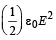(ε0 : permittivity of free space, Eelectric field)

Detailed Solution for Test: Single Correct MCQs: Units and Measurements | JEE Advanced - Question 1

Note : Hererepresents energy per unit volume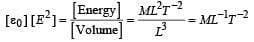Test: Single Correct MCQs: Units and Measurements | JEE Advanced - Question 2

### A quantity X is given by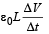where ∈0 is the permittivity of the free space, L is a length, ΔV is a potential difference and Δt is a time interval. The dimensional formula for X is the same as that of

Detailed Solution for Test: Single Correct MCQs: Units and Measurements | JEE Advanced - Question 2

Dimensionally ε0L= Capacitance (c)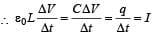Test: Single Correct MCQs: Units and Measurements | JEE Advanced - Question 3

### A cube has a side of length 1.2 × 10–2m. Calculate its volume.

Detailed Solution for Test: Single Correct MCQs: Units and Measurements | JEE Advanced - Question 3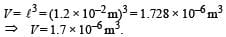Test: Single Correct MCQs: Units and Measurements | JEE Advanced - Question 4

Pressure depends on distance as,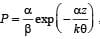, where α, β are constants, z is distance, k is Boltzman’s constant and θ is temperature. The dimension of β are

Detailed Solution for Test: Single Correct MCQs: Units and Measurements | JEE Advanced - Question 4

Unit of k is joules per kelvin or dimensional formula of k is [ML2T–2 θ -1] Note : The power of an exponent is a number.

Therefore, dimensionally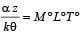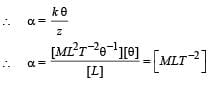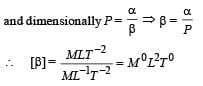Test: Single Correct MCQs: Units and Measurements | JEE Advanced - Question 5

A wire of length ℓ = 6 ± 0.06 cm and radius r = 0.5 ± 0.005 cm and mass m = 0.3 ± 0.003 gm. Maximum percentage error in density is

Detailed Solution for Test: Single Correct MCQs: Units and Measurements | JEE Advanced - Question 5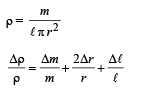Putting the values Δℓ = 0.06 cm, ℓ = 6 cm; Δr = 0.005 cm; r = 0.5 cm,
m = 0.3 gm; Δm = 0.003 gm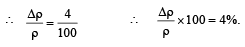Test: Single Correct MCQs: Units and Measurements | JEE Advanced - Question 6

Which of the following set have different dimensions?

Detailed Solution for Test: Single Correct MCQs: Units and Measurements | JEE Advanced - Question 6

Electric flux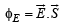∴ Dimensionally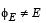Test: Single Correct MCQs: Units and Measurements | JEE Advanced - Question 7

In a screw gauge, the zero of mainscale coincides with fifth division of circular scale in figure (i). The circular division of screw gauge are 50. It moves 0.5 mm on main scale in one rotation. The diameter of the ball in figure (ii) is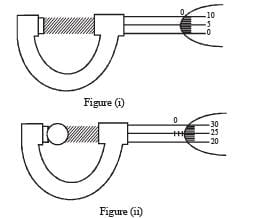Detailed Solution for Test: Single Correct MCQs: Units and Measurements | JEE Advanced - Question 7

Least count = 0.5/50 = 0.01mm

Zero error = 5 × L.C = 5 × 0.01 mm = 0.05 mm
Diameter of ball = [Reading on main scale] + [Reading on circular scale × L . C] – Zero error = 0.5 × 2 + 25 × 0.01 – 0.05  = 1.20 mm

Test: Single Correct MCQs: Units and Measurements | JEE Advanced - Question 8

A student performs an exper iment for determination of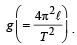The error in length ℓ is Δℓ and in time T is ΔT and n is number of times the reading is taken. The measurement of g is most accurate for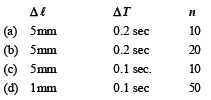Detailed Solution for Test: Single Correct MCQs: Units and Measurements | JEE Advanced - Question 8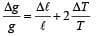Δℓ and ΔT are least and number of readings are maximum in option (d), therefore the measurement of g is most accurate with data used in this option.

Test: Single Correct MCQs: Units and Measurements | JEE Advanced - Question 9

A student performs an experiment to determine the Young's modulus of a wire, exactly 2 m long, by Searle's method. In a particular reading, the student measures the extension in the length of the wire to be 0.8 mm with an uncertainty of ± 0.05 mm at a load of exactly 1.0 kg. The student also measures the diameter of the wire to be 0.4 mm with an uncertainty of ± 0.01 mm. Take g = 9.8 m/s2 (exact). The Young's modulus obtained from the reading is

Detailed Solution for Test: Single Correct MCQs: Units and Measurements | JEE Advanced - Question 9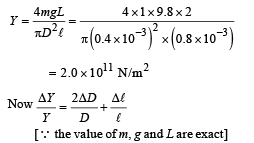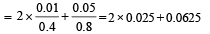= 0.05 + 0.0625 = 0.1125
⇒ ΔY = 2 × 1011 × 0.1125 = 0.225 × 1011
= 0.2 × 1011 N/m2

Note: We can also take value of y from options given without calculating it as it is same in all options.

∴ Y = ( 2 ± 0.2) × 1011 N/m2

Test: Single Correct MCQs: Units and Measurements | JEE Advanced - Question 10

Students I, II and III perform an experiment for measuring the acceleration due to gravity (g) using a simple pendulum.
They use different lengths of the pendulum and /or record time for different number of oscillations. The observations are shown in the table.

Least count for length = 0.1 cm

Least count for time = 0.1 s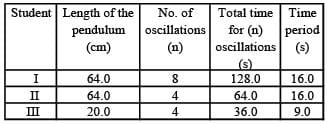If EI, EII and EIII are the percentage errors in g, i.e.,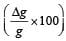for students I, II and III, respectively, then

Detailed Solution for Test: Single Correct MCQs: Units and Measurements | JEE Advanced - Question 10

The time period of a simple pendulum is given by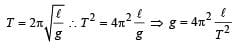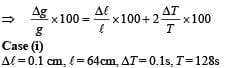Test: Single Correct MCQs: Units and Measurements | JEE Advanced - Question 11

A vernier calipers has 1 mm marks on the main scale. It has 20 equal divisions on the Vernier scale which match with 16 main scale divisions. For this Vernier calipers, the least count is

Detailed Solution for Test: Single Correct MCQs: Units and Measurements | JEE Advanced - Question 11

20 divisions on the vernier scale = 16 divisions of main scale

∴ 1 division on the vernier scale

= 16/20 divisions of main scale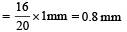We know that least count = 1MSD – 1VSD
= 1 mm – 0.8 mm  = 0.2 mm

Test: Single Correct MCQs: Units and Measurements | JEE Advanced - Question 12

Th e den sity of a solid ball is to be deter mined in an experiment. The diameter of the ball is measured with a screw gauge, whose pitch is 0.5 mm and there are 50 divisions on the circular scale. The reading on the main scale is 2.5 mm and that on the circular scale is 20 divisions. If the measured mass of the ball has a relative error of 2 %, the relative percentage error in the density is

Detailed Solution for Test: Single Correct MCQs: Units and Measurements | JEE Advanced - Question 12

Diameter D = M.S.R. + (C.S.R) × L.C.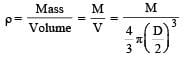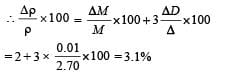Test: Single Correct MCQs: Units and Measurements | JEE Advanced - Question 13

In the determination of Young’s modulus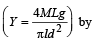using Searle’s method, a wire of length L = 2 m and diameter d = 0.5 mm is used. For a load M = 2.5 kg, an extension l = 0.25 mm in the length of the wire is observed. Quantities d and l are measured using a screw gauge and a micrometer, respectively. They have the same pitch of 0.5 mm. The number of divisions on their circular scale is 100. The contributions to the maximum probable error of the Y measurement

Detailed Solution for Test: Single Correct MCQs: Units and Measurements | JEE Advanced - Question 13

The maximum possible error in Y due to l and d are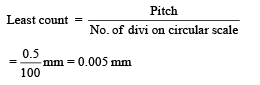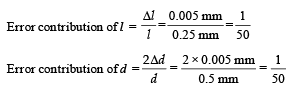Test: Single Correct MCQs: Units and Measurements | JEE Advanced - Question 14

The diameter of a cylinder is measured using a Vernier callipers with no zero error. It is found that the zero of the Vernier scale lies between 5.10 cm and 5.15 cm of the main scale. The Vernier scale has 50 divisions equivalent to 2.45 cm. The 24th division of the Vernier scale exactly coincides with one of the main scale divisions. The diameter of the cylinder is

Detailed Solution for Test: Single Correct MCQs: Units and Measurements | JEE Advanced - Question 14

Reading = M.S.R + No of division of V.S matching the main scale division (1MSD – 1VSD)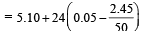= 5.124 cm    Option (b) is correct.

Test: Single Correct MCQs: Units and Measurements | JEE Advanced - Question 15

There are two Vernier calipers both of which have 1 cm divided into 10 equal divisions on the main scale. The Vernier scale of one of the calipers (C1) has 10 equal divisions that correspond to 9 main scale divisions. The Vernier scale of the other caliper (C2) has 10 equal divisions that correspond to 11 main scale divisions. The readings of the two calipers are shown in the figure. The measured values (in cm) by calipers C1 and C2, respectively, are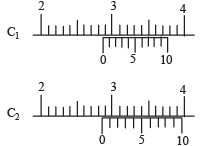Detailed Solution for Test: Single Correct MCQs: Units and Measurements | JEE Advanced - Question 15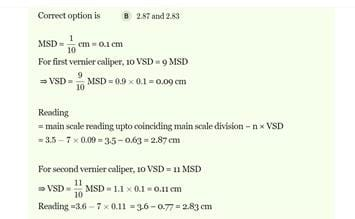## JEE Foundation

99 videos|291 docs|212 tests
 Use Code STAYHOME200 and get INR 200 additional OFF Use Coupon Code
Information about Test: Single Correct MCQs: Units and Measurements | JEE Advanced Page
In this test you can find the Exam questions for Test: Single Correct MCQs: Units and Measurements | JEE Advanced solved & explained in the simplest way possible. Besides giving Questions and answers for Test: Single Correct MCQs: Units and Measurements | JEE Advanced, EduRev gives you an ample number of Online tests for practice

## JEE Foundation

99 videos|291 docs|212 tests